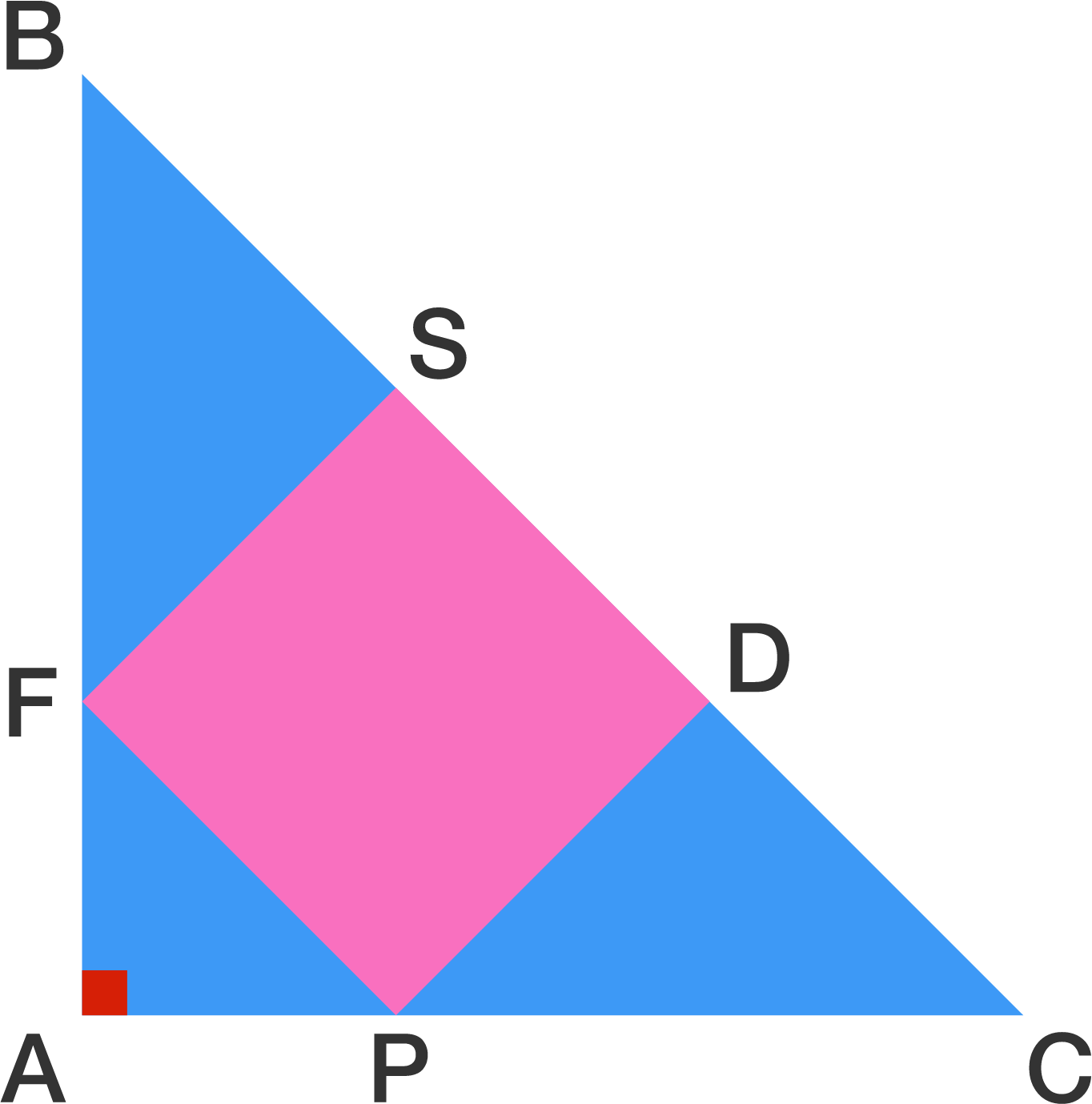# Fit just nicely

Geometry Level 1$ABC$ is an isosceles right triangle with $AB = AC = 6$.

Given that $SDPF$ is a square, find the area of the square.

×